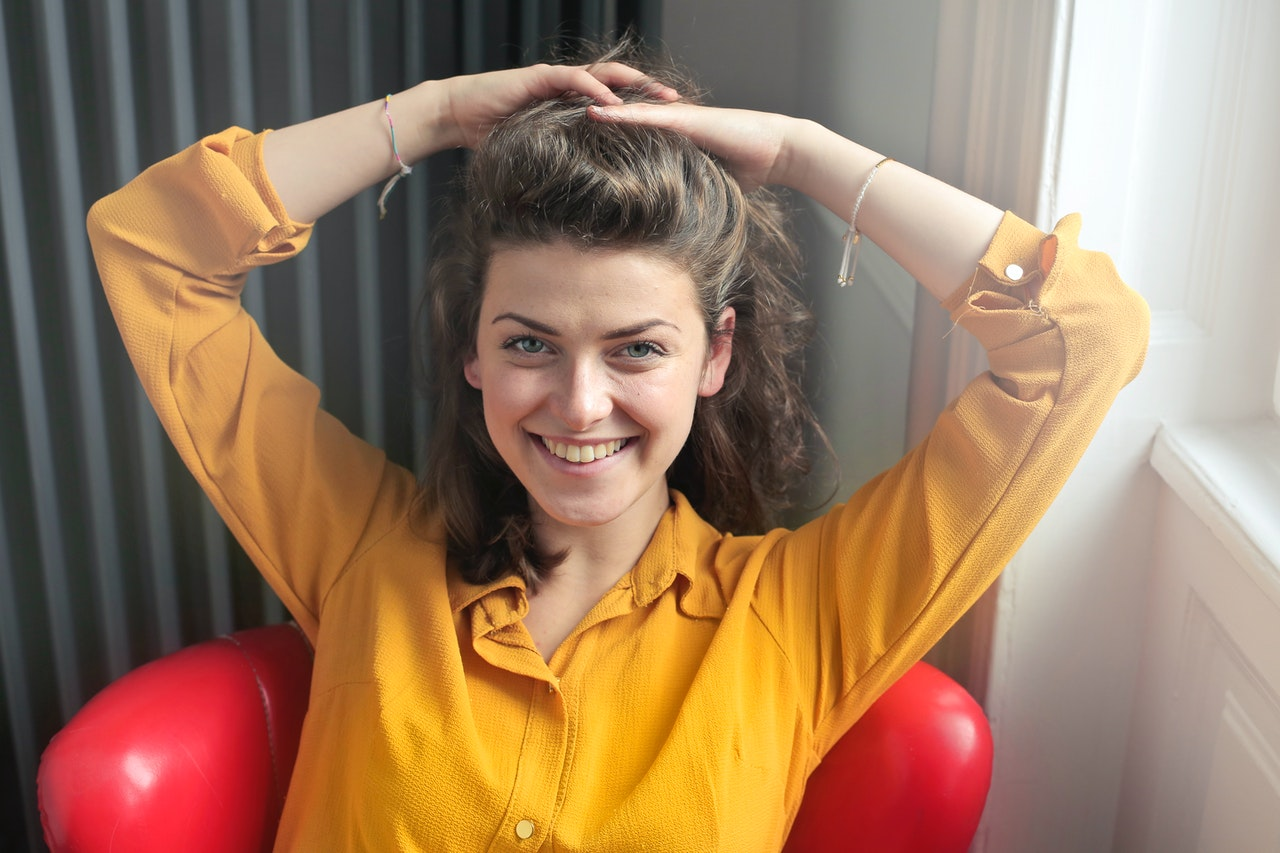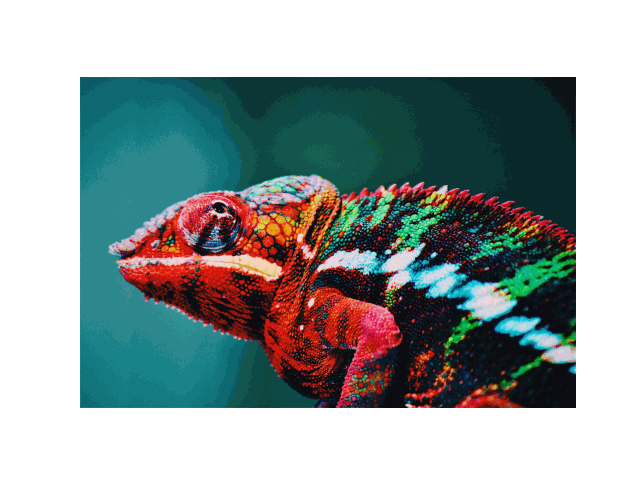# pixel-shuffle

## the idea

There are two primary ways to do image morphing. The simplest method works by interpolating between two images (i.e., one fades out and the other fades in). The other involves stretching the features of the image and distorting the colors to blend between two images.

This project differs from both in that it preserves the color of every single pixel in the original image. Instead of distorting colors, it rearranges all the pixels to produce the second image. The result is a picture that has the form and structure of the second image and the colors of the first image.

To the best of my knowledge, this project is the first publicly available implementation of this approach.

## the math

We have two `m` by `n` images, each of which can be interpreted as a set of `mn` RGB tuples. For each tuple in the first set, we want to find the tuple in the second set that is most similar. To judge what is most similar, we minimize Euclidean distance (but it would be interesting to experiment with other metrics as well). Given any tuple from the first set and any tuple from the second set, we can associate with the pair a “cost”, given by the Euclidean distance. Another way to think of this setup is that we have a complete bipartite graph, where the tuples of one set are on one side and the tuples of the other set are on the other side. We assign each edge a weight based on the cost associated with the pair of endpoints.

Finding the optimal assignment of pixels to construct the image morph is equivalent to finding a minimum weight bipartite matching in this graph. This is exactly the balanced assignment problem. Luckily for us, included in the SciPy library is an efficient method for solving this problem.

## the result!